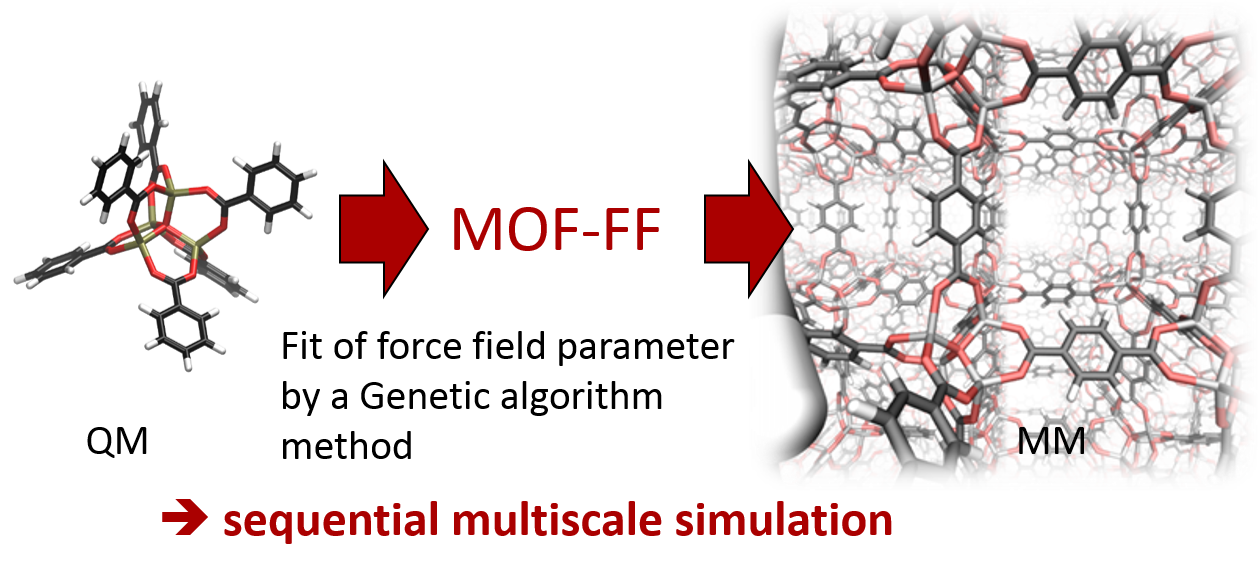### MOF-FF

MOF-FF is a first principles parametrized force field for metal-organic frameworks. The parameters are adjusted to reproduce structure and PES curvature information of non-periodic model systems, computed on dispersion corrected hybrid-DFT level.The MOF-FF bond term is taken from MM3:MOF-FF is an ab initio parameterized FF especially designed for the application on MOFs. It was developed by the CMC group and is used among others things for screening of isoreticular isomers in the reverse topological approach. Its parameterization is based on the bottom-up approach relying on the fit of geometric and curvature reference data of building blocks in redundant internal coordinates. The reference data is obtained by density functional theory calculations.

#### Motivation

MOFs are a relative new class of porous materials, where inorganic parts are connected by organic linkers. Due to the large unit cells with many transition metals accurate ab initio methods - like periodic DFT calculations - are numerically often too demanding, especially if many different configurations/isomers have to be addressed. A less demanding alternative are non ab initio, approximate methods like molecular mechanics (MM) or classical force fields (FF).

The accuracy of these methods depends crucially on the quality of their parameterization. Reliable predictions are only possible if the FF has been parameterized in an adequate way and is valid in the addressed area of the potential energy surface (PES).

For the organic linkers a huge variety of well parameterized FFs exists, but they do not describe the metal-ligand interaction, which is crucial for the overall quality of the FF. MOF-FF provides a systematic approach for the generation of accurate FFs for MOFs or related coordination compounds directly from DFT reference data.

#### Energy Terms

The energy expression of MOF-FF is the sum of five additive terms:which should be explained in the following.

The expression for the vdW interactions is a dispersion-damped Buckingham potential. The parametrization is taken from Allinger et. al. . Note that the so called 1-2 and 1-3 interactions between two bond partners are switched off in MOF-FF.

For the expression of the electrostatic interactionsit is required to know the charge of every atom in the system. In MOF-FF, Gaussian-type charge distributions are used. The advantage is that electrostatic interactions for high charges at close distances are damped. Consequently, all atom-atom interactions are included in the Coulomb sum. The charges are taken from a fit to the electrostatic potential by the scheme introduced by Merz and Kollmann .

##### Stretches

For regular bond stretches a quartic potential in the MM3 formulation .In addition also a Morse potential, is available.withas inharmonicity parameter defined as,

whereis the dissociation energy of the bond. The Morse potential is used for softer coordination bonds with a predefined dissociation energy.

##### In-plane Bends

For in-plane bending again two potentials are available. The first one is an anharmonic corrected MM3 potential (six order polynomial form) with fixed anharmonic contributions.is the bond angle,is the reference bond angle, andis the force constant.

In addition also a special Fourier term is available.withas energy barrier,as phase shift andas multiplicity. It is used for coordination complexes with coordination numbers beyond four or in case of square planar coordination, where polynomial angle bending terms with a single minimum cannot be used. Note that for these terms 1-3 vdW interactions are included in order to prevent low angles due to atomic repulsion.

##### Torsions

Torsions are treated in MOF-FF using a Fourier series term up to the multiplicity.with the height of the barrierand the dihedral angle.

##### Out-of-plane bends

Out-of-plane bending at trigonal centers is modelled by an out-of-plane bending term, using the Wilson-Decius definition of the out-of-plane angle. Therefore, a simple harmonic potential with the force constantis used.##### Coupling Terms

In addition to these regular terms, some cross terms, sometimes called off-diagonal or coupling terms, are included in MOF-FF. They couple different DOFs to each other and describe for example, how the force constant of a bond stretchdepends on the change of a neighboring angle bending or bond stretch. In MOF-FF only stretch-stretchand stretch-bendcouplings for three connected atoms A-B-C are implemented.,.

The distancerefers to the distance A-B,refers to the distance B-C and the angleto the angle A-B-C. The reference values,andare equal to the reference values given in the corresponding stretch and bending potentials.,andare the coupling force constants.